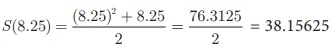Home | | Maths 10th Std | Special cases of function

# Special cases of function

There are some special cases of a function which will be very useful. We discuss some of them below (i) Constant function (ii) Identity function (iii) Real – valued function

Special cases of function

There are some special cases of a function which will be very useful. We discuss some of them below

(i) Constant function

(ii) Identity function

(iii) Real – valued function

## (i) Constant function

A function f : A B is called a constant function if the range of f contains only one element. That is, f (x ) = c , for all x A and for some fixed c B.

### Illustration 16

From Fig.1.37, A = {a,b,c,d} and B = {1, 2, 3} and  f  = {(a, 3),(b, 3),(c, 3),(d, 3)} . Since,  f (x) = 3 for every x A ,  Range of  = {3} , is a constant function.## (ii) Identity function

Let A be a non–empty set. Then the function f: A A defined by f (x) = x for all x A is called an identity function on A and is denoted by IA.### Illustration 17

If A = {a,b,c} then f=IA = {(a,a),(b,b),(c,c)} is an identity function on A.

## (iii) Real valued function

A function f: A B is called a real valued function if the range of f is a subset of the set of all real numbers R . That is, f (A) R.

Example 1.17

Let f  be a function from R  to R defined by f (x) = 3x − 5 . Find the values of a and b given that (a, 4) and (1, b) belong to f.

Solution f (x) = 3x – 5 can be written as f   = {(x, 3x – 5) | x R}

(a, 4) means the image of a is 4. That is, f (a) = 4

3a – 5 = 4       a = 3

(1, b) means the image of 1 is b.

That is, f (1) = b b = −2

3(1) – 5 = b Þ b = –2

Example 1.18 The distance S (in kms) travelled by a particle in time ‘t’ hours is given by S(t) = [ t2 + t ]/2. Find the distance travelled by the particle after

(i) three and half hours.

(ii) eight hours and fifteen minutes.

Solution The distance travelled by the particle in time t hours is given by(i) t = 3.5 hours. ThereforeThe distance travelled in 3.5 hours is 7.875 kms.

t = 8.25 hours. ThereforeThe distance travelled in 8.25 hours is 38.16 kms, approximately.

### Example 1.19

If the function f : RR is defined by,

then find the values of

(i) f (4)

(ii) f (-2)

(iii) f (4) + 2f (1)

(iii) [f (1) + 3f (4)] / f (-3)

### Solution

The function f is defined by three values in intervals I, II, III as shown by the sideFor a given value of x = a , find out the interval at which the point a is located, there after find f (a) using the particular value defined in that interval.

(i) First, we see that, x = 4 lie in the third interval.

Therefore, f (x) = 3x − 2 ; f (4) = 3(4) – 2 = 10

(ii) x = −2 lies in the second interval.

Therefore, f (x) = x2 – 2 ; f (−2) = (−2)2 – 2 = 2

(iii) From (i), f (4) = 10 .

To find f (1), first we see that x = 1 lies in the second interval.

Therefore, f (x) = x2 – 2 f (1) = 12 – 2 = −1

So, f (4) + 2f (1) = 10 + 2(−1) = 8

(iv) We know that f (1) = -1 and f (4) = 10.

For finding  f (-3), we see that = −3 , lies in the first interval.

Therefore, f (x) = 2x + 7 ; thus, f (−3) = 2(−3) + 7 = 1

HenceTags : Definition, Illustration, Example, Solution | Mathematics , 10th Mathematics : UNIT 1 : Relation and Function
Study Material, Lecturing Notes, Assignment, Reference, Wiki description explanation, brief detail
10th Mathematics : UNIT 1 : Relation and Function : Special cases of function | Definition, Illustration, Example, Solution | Mathematics